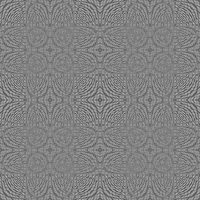Monday, April 14, 2008

Monday Morning Algorithm 15: Building a Noiselet Basis and Measurement MatrixFor today's algorithm, Laurent Duval, a reader of this blog, decided to implement the noiselet basis of Coifman, Geshwind and Meyer  and share it. This basis has the particularity of being incoherent with the Haar Basis and therefore it is of particular interest in imaging in the compressed sensing framework (since pixels are Heaviside/Haar functions). It was mentioned before here and here. The script is available here but is also part of the Monday Morning Algorithm page and the Compressed Sensing code page as well.

function [noiselet_Matrix] = Build_Noiselets(n_Power)

% [noiselet_Matrix] = Build_Noiselets(n_Power)
% Built an orthonormal noiselet matrix with 2^n_Power
% In: n_Power (integer); default: n_Power = 5 (n_Sample = 2^n_Power = 32)
% Out: noiselet_Matrix (complex array); default (no outpu): display real and
% imaginery parts
% Example: noiselet_Matrix_1024 = Build_Noiselets(10);
%
% Comments: no optimization at all, suggestions welcome
% Creation: 2008/04/10
% Update: 2008/04/13
%
% Author: Laurent Duval
% URL: http://www.laurent-duval.eu

if nargin == 0
n_Power = 5;
end
n_Sample = 2^n_Power;

noiselet_Matrix = zeros(n_Sample,2*n_Sample-1);
noiselet_Matrix(:,1) = 1;
coef1 = 1 - i;
coef2 = 1 + i;
vect_Fill = zeros(n_Sample/2,1);
for i_Col = 1:n_Sample-1
vect_2x = [noiselet_Matrix(1:2:n_Sample,i_Col);vect_Fill];
vect_2x_1 = [vect_Fill;noiselet_Matrix(1:2:n_Sample,i_Col)];
noiselet_Matrix(:,2*i_Col) = coef1*vect_2x + coef2*vect_2x_1;
noiselet_Matrix(:,2*i_Col+1) = coef2*vect_2x + coef1*vect_2x_1;
end
noiselet_Matrix = 1/n_Sample*noiselet_Matrix(:,n_Sample:2*n_Sample-1);

if nargout == 0
figure(1)
subplot(1,2,1)
imagesc(real(noiselet_Matrix))
xlabel('Real part')
subplot(1,2,2)
imagesc(imag(noiselet_Matrix))
xlabel('Imaginery part')
end

Laurent is making some anagram on noiselets in his blog. As usual now, the disclaimer for using this script is listed here.